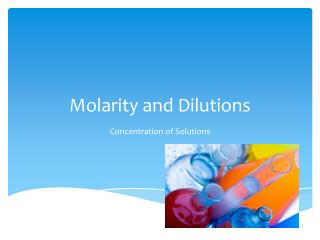Download PresentationMolarity and Dilutions

Molarity and Dilutions - PowerPoint PPT Presentation

Molarity and Dilutions. Concentration of Solutions. Molarity. Molarity – Measurement of the concentration of a solution (how much solute is in a volume of solution) Molarity (M) is equal to the number of moles of solute (n) per liter of solution.I am the owner, or an agent authorized to act on behalf of the owner, of the copyrighted work described.
Download PresentationMolarity and Dilutions

Download Policy: Content on the Website is provided to you AS IS for your information and personal use and may not be sold / licensed / shared on other websites without getting consent from its author.While downloading, if for some reason you are not able to download a presentation, the publisher may have deleted the file from their server.

- - - - - - - - - - - - - - - - - - - - - - - - - - E N D - - - - - - - - - - - - - - - - - - - - - - - - - -
Presentation Transcript
1. Molarity and Dilutions Concentration of Solutions

2. Molarity Molarity – Measurement of the concentration of a solution (how much solute is in a volume of solution) Molarity (M) is equal to the number of moles of solute (n) per liter of solution Molarity (M) = moles of solute / liters of solution M = mol / L

3. Molarity Be careful not to get molarity and moles mixed up. Moles measures the amount of material you have. Molarity measures the concentration of the material.

4. Molarity Suppose we had 1.oo mole of sucrose and proceeded to mix it into some water. It would dissolve and make sugar water. We keep adding water, dissolving and stirring until all the solid was gone. We then made sure that when everything was well-mixed, there was exactly 1.00 liter of solution. What would be the molarity of the solution? M = 1.00 mole / 1.00 liter = 1.00 mol/L or 1.00 M Often, instead of writing the units as mol/L, a capital M is used (M = mol/L)

5. Molarity Examples 1. Calculate the molarity of a solution prepared by mixing 1.5 g of NaCl in 500.0 mL of water First calculate the moles of solute in the solution: 1.5 grams NaCl 1 molNaCl 58.443 grams NaCl = 0.026 molNaCl Next convert mL to L: 0.500 L Last, plug the appropriate values into the correct variables in the equation: M = n / V = 0.026 mol / 0.500 L = 0.052 mol/L or 0.052 M

6. Molarity Examples 2. Calculate the mass of NaOH needed to prepare 1.00 L of a 1.50 M solution. To do this, the formula for molarity needs to be rearranged in order to solve for the number of moles: M = moles / liters moles = M x liters Numbers are then plugged into rearranged formula: moles = (1.50 mol/L) x (1.00 L) = 1.50 molNaOH We then find the grams in the 1.50 mol of NaOH by using dimensional analysis 1.50 molNaOH 39.997 grams NaOH 1 molNaOH = 59.9955 grams NaOH = 60.0 grams NaOH

7. Molarity Examples 3. 965 grams of NaOH is used in preparing a 1.5M solution. What volume of solution can be made? First calculate the moles of solute in the solution: 965 grams NaOH 1 molNaOH 39.997 grams NaOH = 24.1 molNaOH We now rearrange the formula for molarity to solve for volume (liters): M = moles / volume volume = moles / M Numbers are then plugged into rearranged formula: volume = (24.1 mol) / (1.5 mol/L) = 16.0666 L = 16 L

8. Your Turn…… • Sea water contains roughly 28.0 g of NaCl per liter. What is the molarity of sodium chloride in sea water? • What is the molarity of 245.0 g of H2SO4 dissolved in 1.00 L of solution? • How many moles of Na2CO3 are there in 10.0 L of 2.0 M solution? • What weight (in grams) of H2SO4 would be needed to make 750.0 mL of 2.00 M solution? • What volume of 18.0 M H2SO4is needed to contain 2.45 g H2SO4?

9. Dilutions • Dilution: The process of decreasing the concentration of a stock solution by adding more solvent to the solution. • The solvent added is usually the universal solvent, known as water. The more solvent you add, the more diluted the solution will get. • - A stock solution is a concentrated solution that will be diluted to a lower concentration for actual use

10. Dilutions • The equation for dilution is • M1V1=M2V2 • stock solution= diluted solution • M1= molarity of the stock solution • M2= molarity of the diluted solution • V1= volume of stock solution • V2= volume of diluted solution • Basically, dilution calculations involve figuring out the final concentration or volume ( depending on what’s given and what’s known) after a volume or concentration has been changed. • In dilution equations, you are given three things and you need to find the fourth component.

11. Dilution Examples 1. If I add 25 mL of water to 125 mL of a 0.150 M NaOH solution, what will the molarity of the diluted solution be? 2. If I add water to 100 mL of a 0.150 M NaOH solution until the final volume is 150 mL, what will the molarity of the diluted solution be?

12. Dilution Examples 1. I have 345 mL of a 1.5 M NaCl solution. If I boil the water until the volume of the solution is 250 mL, what will the molarity of the solution be? 2. How much 0.05 M HCl solution can be made by diluting 250 mL of 10 M HCl?